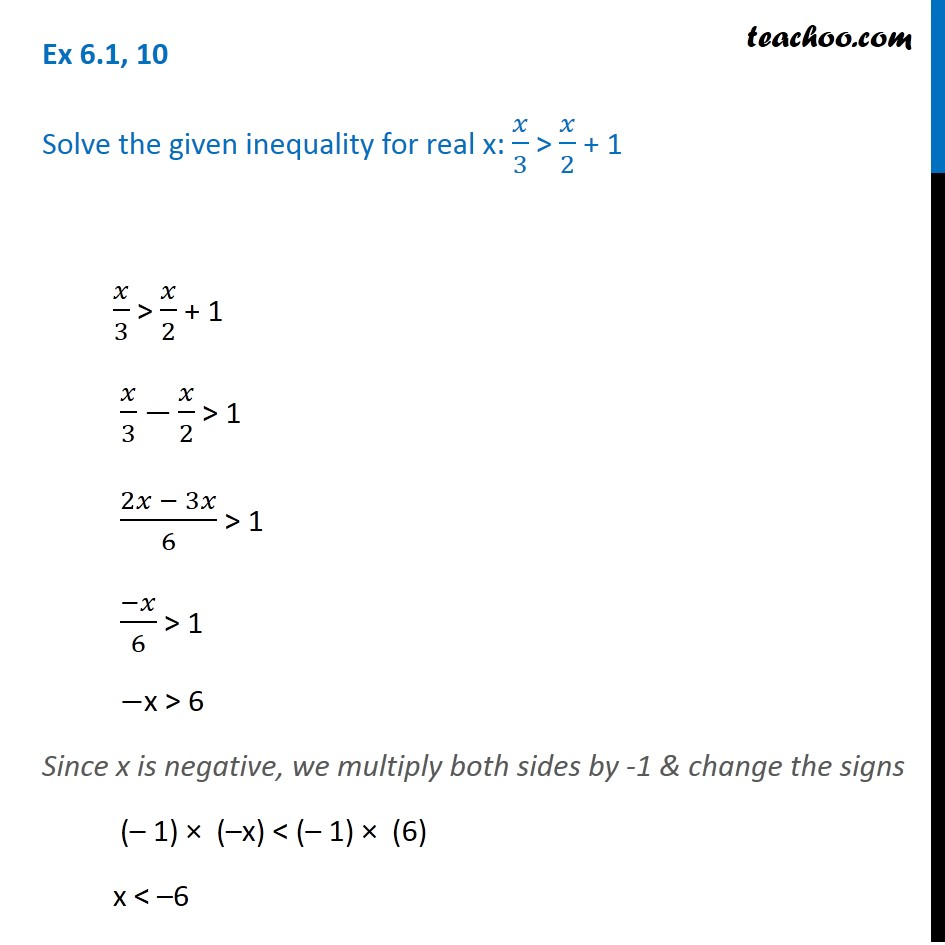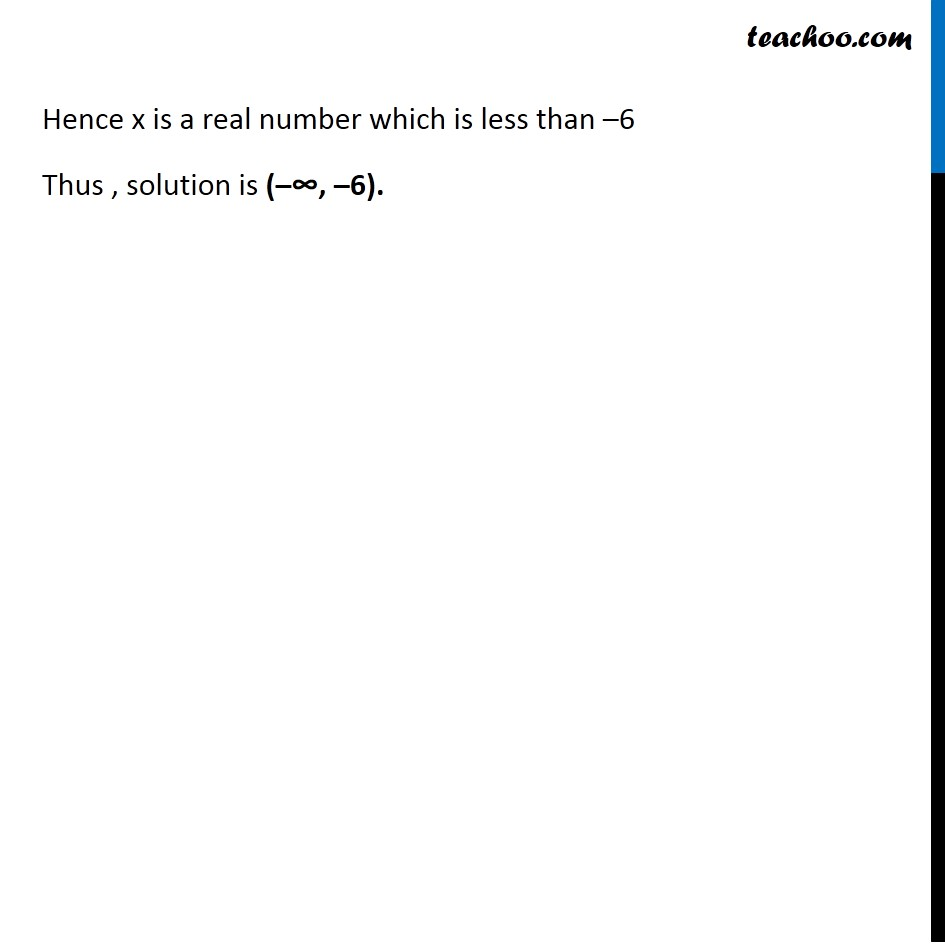1. Chapter 6 Class 11 Linear Inequalities (Term 2)
2. Serial order wise
3. Ex 6.1

Transcript

Ex 6.1, 10 Solve the given inequality for real x: 𝑥/3 > 𝑥/2 + 1 𝑥/3 > 𝑥/2 + 1 𝑥/3 −𝑥/2 > 1 (2𝑥 − 3𝑥)/6 > 1 (−𝑥)/6 > 1 −x > 6 Since x is negative, we multiply both sides by -1 & change the signs (– 1) × (–x) < (– 1) × (6) x < –6 C Hence x is a real number which is less than –6 Thus , solution is (–∞, –6).